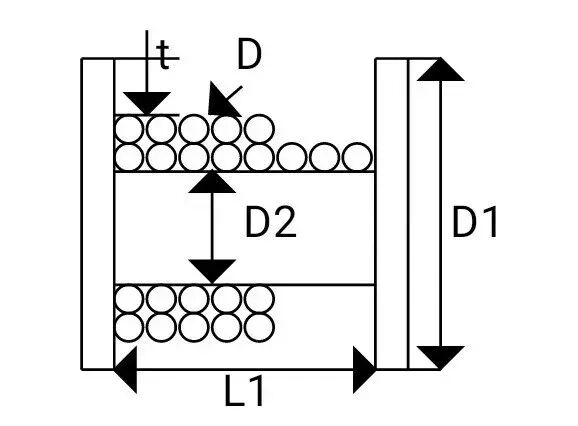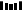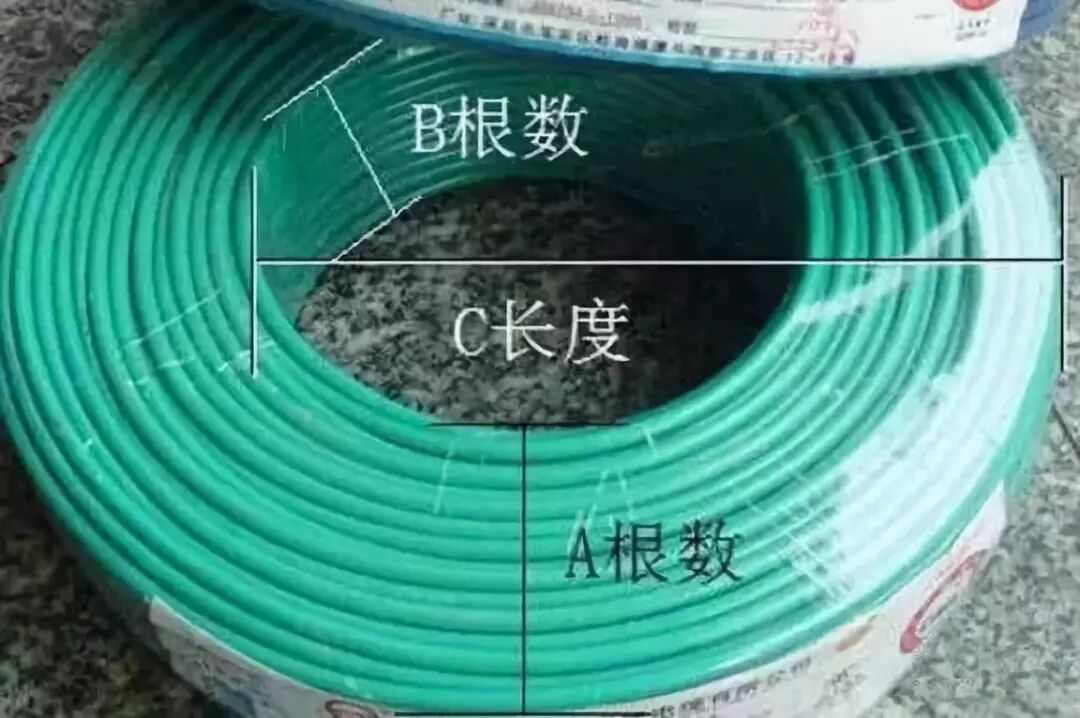欢迎来到浙江人民线缆制造官方网站！ 官方微信　|　收藏我们　|　网站地图　|线缆网首页 线缆产品 合作案例 线缆百科 线缆问答 线缆商城 人民线缆简介 联系我们

### 特种电缆### 降包装成本！一种新的电缆盘装线长度计算方法（亲测有效

1）电缆盘自身的稳定性，即包装盘在装电线电缆产品时，包装盘不变形，经得起吊卸；

2）电缆盘的肚径要满足被包装电缆产品的弯曲半径；

3）电缆盘要能装下被包装电缆特定长度的产品；

1π/4*D?*L=π/4*[(D?-2t)2-D?2]

L=[(D?-2t)2- D?2]/ D?·········

L=0.87*[（D?-2t）2- D?2]/ D?·········

2

n=L?/D×0.95(系数0.95考虑实际情况排线不齐)·········

P=(D?-D?-2*t)/(2*D)·········

L’= p0π(D?+2D*x)dx=π(D?*x+D*2)P0

=π(D?*p+D*P?)·········

L=n* L’=π*n*( D?*p+D*P?)/1000

=π*n*p*( D?+D*P)/1000·········

3

L”=π[D?+(D?-D?-2*t)/2]

=π(D?+D?-2*t)/2·········

n=L?/D×0.95·········

(系数0.95考虑实际情况排线不齐，计算结果取整数部分值）

P=(D?-D?-2*t)/(2*D)·········?

（计算结果取整数部分值)

L=n*p* L”

=π* n*p*(D?+D?-2*t)/2000·········?

D?+D*P与(D?+D?-2*t)/2

=2*P*D+D?·········?

B=(D?+2*P*D+D?)/2=(2*D?+2*P*D)/2

=D?+D*P=A

4

L”=π[D?+(D?-D?-2*t)/2]

= π(D?+D?-2*t)/2·········?

n=L?/a×0.95·········?

(系数0.95考虑实际情况排线不齐，计算结果取整数部分值)

P=(D?-D?-2*t)/(2* b)·········?

（计算结果取整数部分值)

L=n*p* L”

=π* n*p*(D?+D?-2*t)/2000·········?

*作者简介：方健银，湖北易缆通模具有限公司总经理兼总工程师；毕业于河南工学院电线电缆制造技术专业；电缆导体25-630mm2节能降耗新工艺“异型线绞合电缆导体及生产方法”发明人（发明专利号：201410338703.5）A根数：横面电线的根数（把电线横着放数出有多少根）

B根数：竖面电线的根数（把电线竖着放数出有多少根）

C长度：外围任意一边到内盘的长度（单位米）

*来源：易缆通线缆模具、缆资讯；如有侵权请联系编辑，转载请注明出处

##### 相关资讯